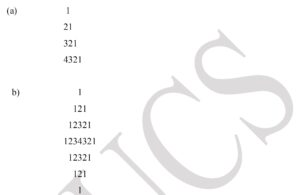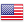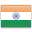Grade "A+" Accredited by NAAC with a CGPA of 3.46
Grade "A+" Accredited by NAAC with a CGPA of 3.46

# Programming using Python

Course ID
CSGE 101
Level
Program
B.Sc. CS (Hons.)
Semester
First
Credits
6.0
Paper Type
Generic Elective
Method
Lecture & Practical

## Unique Paper Code: 32345104

This course is designed as the first course that introduces computers and programming to non-Computer Science students. The course focuses on the use of computer and programming to solve problems of different domains. It also introduces the concept of object- oriented programming.

## Learning Outcomes:

At the end of the course, students should be able to:

• Describe the components of a computer and notion of an algorithm.
• Apply suitable programming constructs and built-in data structures to solve a problem.
• Develop, document, and debug modular python programs.
• Use classes and objects in application programs and visualize data.

## Course Contents

Unit 1
Unit 2
Unit 3
Unit 4
Unit 5
Unit 6

Unit 1

Computer Fundamentals and Problem Solving: Basic Computer Organization: CPU, memory, I/O Units. Problem solving using computer, notion of an algorithm.

Unit 2

Introduction to Python Programming: Python interpreter/shell, indentation; identifiers and keywords; literals, numbers, and strings; operators (arithmetic operator, relational operator, Boolean operator, assignment, operator, ternary operator and bitwise operator) and expressions.

Unit 3

Creating Python Programs: Input and output statements, defining functions, control statements (conditional statements, loop control statements, break, continue and pass, exit function.), default arguments, errors and exceptions.

Unit 4

Strings and Lists: String class, built-in functions for string, string traversal, string operators and operations; Lists creation, traversal, slicing and splitting operations, passing list to a function.

Unit 5

Object Oriented Programming: Introduction to Classes, Objects and Methods, Standard Libraries, File handling through libraries.

Unit 6

Built-in data structures: Tuples, sets, dictionary, stacks, and queues; searching and sorting.

### Practicals

#### Lab List 1

1. Execution of expressions involving arithmetic, relational, logical, and bitwise operators in the shell window of Python IDLE.
2. Write a Python function to produce the outputs such as:1. Write a Python program to illustrate the various functions of math module.
2. Write a Python program to produce a table of sins, cosines and tangents. Make a variable x in range from 0 to 10 in steps of 0.2. For each value of x, print the value of sin(x), cos(x) and tan(x).
3. Write a program that reads an integer value and prints “leap year” or “not a leap year”
4. Write a menu driven program to calculate the area of given building. Accept suitable inputs and use suitable assertions.
5. Write a Python function that takes a number as an input from the user and computes its factorial.
6. Write a Python function to generate the Fibonacci sequence till a given number “n”.
7. Write a function that takes a number as an input and finds its reverse and computes the sum of its digits.
8. Write a function that takes two numbers as input parameters and returns their least common multiple.

#### Lab List 2

1. Write a function that takes a number as an input and determine whether it is prime or not.
2. Write a function that finds the sum of the
a) first n odd terms
b) first n even terms
c) 1, 2, 4, 3, 5, 7, 9, 6, 8, 10, 11, 13.. till n-th term
3. Write a Python function that takes a string as an input from the user and determines whether it is palindrome or not.
4. Write a function that takes a sentence as input from the user and calculates the frequency of each letter. Use a variable of dictionary type to maintain the count.
5. Write a Python function that prints a dictionary where the keys are numbers between 1 and 5 and the values are cubes of the keys.
6. Consider a tuple t1=(1,2,5,7,9,2,4,6,8,10). Write a program to perform following operations:
a. Print half the values of tuple in one line and the other half in the next line.
b. Print another tuple whose values are even numbers in the given tuple.
c. Concatenate a tuple t2=(11,13,15) witht1.
d. Return maximum and minimum value from this tuple.
7. Write a function called “check_duplicates” that takes a list and returns true if there is any element that appears more than once. Also find the frequency of that element. The original list should not be modified.
8. Write a program to implement a class for finding area and perimeter of a rectangle. Write constructor, destructor, and functions for calculating area and perimeter.
9. Write a menu driven program to perform the following functions on strings:
a. Find the length of string
b. Return maximum of three strings
c. Accept a string and replace every successive character with ‘#’ Example- For Given string ‘Hello World’ returned string is ‘H#l#o W#r#d’.
d. Find number of words in the given string
10. Write a Python program to perform the following using list:
a. Check if all elements in list are numbers or not
b. If it is a numeric list, then count number of odd values in it
c. If list contains all Strings, then display largest String in the list
d. Display list in reverse form
e. Find a specified element in list
f. Remove the specified element

#### Lab List 3

1. Usage of Python debugger tool-pydb and PythonTutor.
2. Implementation of Linear and binary search techniques
3. Implementation of selection sort, insertion sort, and bubble sort techniques

#### Text Books

Guttag, J.V. (2016). Introduction to computation and programming using Python. 2nd edition. MIT Press.
Taneja, S., Kumar, N. (2018). Python Programming- A modular Approach. Pearson Education India.

Kamthane, A. N., & Kamthane, A.A. (2017) Programming and Problem Solving with Python, McGraw Hill Education.
Liang, Y. D. (2013). Introduction to Programming using Python. Pearson Education.

#### Teaching Learning Process

Use of ICT tools in conjunction with traditional class room teaching methods
Interactive sessions
Class discussions

#### Assessment Methods

Written tests, assignments, quizzes, presentations as announced by the instructor in the class

#### Keywords

Computer Hardware Organization, Problem solving for computer programming, Object oriented programming, Python.

Disclaimer: Details on this page are subject to change as per University of Delhi guidelines. For latest update in this regard please refer to the University of Delhi website here.Englishहिन्दी